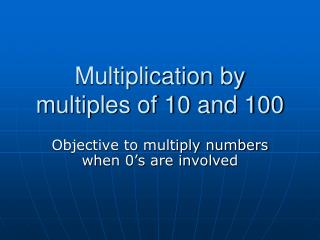DownloadDownload PresentationMultiplication by multiples of 10 and 100

# Multiplication by multiples of 10 and 100

Télécharger la présentation## Multiplication by multiples of 10 and 100

- - - - - - - - - - - - - - - - - - - - - - - - - - - E N D - - - - - - - - - - - - - - - - - - - - - - - - - - -
##### Presentation Transcript

1. Multiplication by multiples of 10 and 100 Objective to multiply numbers when 0’s are involved

2. Starter • What is the rule for multiplying a whole number by 10? • Write down the number and add a zero • What is the rule for multiplying a whole number by 100? • Write down the number and add two zeros

3. 47 X 10 23 X 100 16 X 100 52 X 10 84 X 10 79 X 100 31 X 100 95 X 1000 58 X 10,000 470 2,300 1,600 520 840 7,900 3,100 95,000 580,000 Calculate the following:

4. Main Activity • Calculate 30 X 40 • Multiply 3 X 4 • 12 • How many zeros in the question? • Two • 30 X 40 = 1200 • OR 3 X 4 = 12 30 X 4 = 120 so 30 X 40 =1200

5. Another example • Calculate 40 X 500 • Multiply 4 by 5 • 20 • How many zeros in the question? • Three • 40 X 500 = 20,000

6. Yet another example • Calculate 700 X 300 • Multiply 7 by 3 • 21 • How many zeros in the question? • Four • 700 X 300 = 210,000

7. 200 X 70 60 X 30 800 X 400 2,000 X 30 14,000 1,800 320,000 60,000 Lets check that we understand

8. The rule-plenary • We are to multiply two numbers that are multiples of powers of ten • Firstly we multiply the two digits together ignoring the zeros • We work out how many zeros in the question • We add the number of zeros to our initial answer